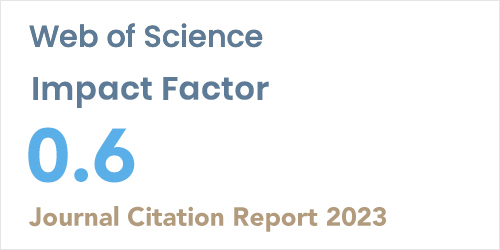ISSN: 1304-7191 | E-ISSN: 1304-7205
Volume: 41 Issue: 4 Year: 2023Degree-Based Invariants of Mycielski Construction: Irregularity, Total Irregularity, Variance
1Dokuz Eylul University, Department of Computer Science, IZMIR
Sigma J Eng Nat Sci 2019; 37(3): 747-754

#### Abstract

The degree-based graph invariants are parameters defined by degrees of vertices. A graph is regular if all of its vertices have the same degree. Otherwise a graph is irregular. To measure how irregular a graph is, graph topological indices were proposed including the irregularity of a graph, total irregularity of a graph, and the variance of the vertex degrees. In this paper, the above mentioned irregularity measures for Mycielski constructions of any underlying graph are considered and exact formulae are derived.